### Home > CALC > Chapter 3 > Lesson 3.3.3 > Problem3-123

3-123.
1. Evaluate each limit. If the limit does not exist, say so but also state if y is approaching positive or negative infinity. Homework Help ✎

1.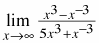2.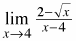3.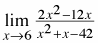4.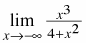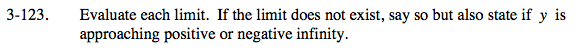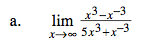As with all limits where x→∞ or x→−∞,compare the highest-power term in the numerator with the highest-power term in the denominator. Be sure to consider coefficients.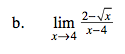$-\lim_{x\rightarrow 4}\left (\frac{\sqrt{x}-2}{x-4} \right ).$

This is Ana's method to find a derivative. If you need more guidance, refer to the hints in problem 3-110 part (b).

Or you could multiply the numerator and the denominator by the conjugate of

$(2-\sqrt{x}).$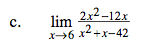When you evaluate at x = 6, there is a 0 in the denominator. Perhaps you can cancel it out. Try factoring.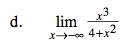Refer to the hint in part (a).